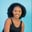Related Tags

numpy
python3

# How to use the np.divide() function for a 2D array in PythonMaria Elijah

## Overview

The Python library NumPy has a method called divide() which can be used to divide two input arrays.

### The numpy.divide() method

The numpy.divide() method divides two input arrays, element-wise (element by element).

Note: A two-dimensional (2D) array can be created using a list of lists in python.

### Syntax

numpy.divide(x1, x2, dtype=None, out=None)


### Parameters

• x1: array_like, represents the input data.
• x2: array_like, represents the input data.
• dtype: This is an optional parameter. It represents the return type of the array.
• out: This is an optional parameter. It represents the alternative output array in which the result is to be placed.

Note: If x1 and x2 have distinct shapes, they must be able to be broadcasted to a common shape for output representation.

### Return value

The numpy.divide() method returns the division of the two input arrays, x1 and x2.

Note: If the out parameter is specified, it returns an array reference to out.

### Code

The following code shows how to divide two-dimensional (2D) arrays using the numpy.divide() method.

# import numpy
import numpy as np
# create a two 2D arrays
x1 = np.array([[2,6,5],[3,4,8]])
x2 = np.array([[1,7,2],[10,9,4]])

# divide the 2D arrays
# and store the result in arr_mul
arr_div = np.divide(x1, x2)

print(arr_div)
Implementing the numpy.divide() function

### Explanation

• Line 2: We import the numpy library.
• Lines 4–5: We create two 2D arrays, x1 and x2.
• Line 9: We use the np.divide() to divide the arrays, x1 and x2. The result is stored in a new variable called arr_div.
• Line 11: We display the result.

RELATED TAGS

numpy
python3

CONTRIBUTORMaria Elijah
RELATED COURSES

View all Courses

Keep Exploring

Learn in-demand tech skills in half the time